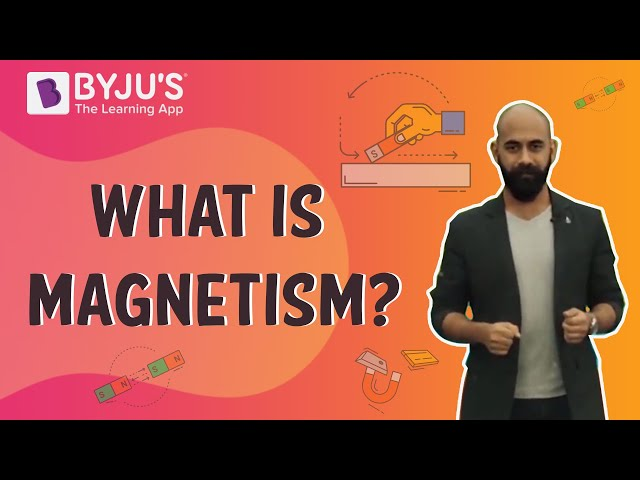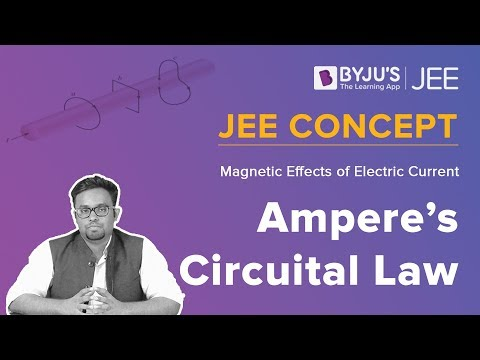# CBSE Class 12 Physics Notes Chapter 4 Moving Charges and Magnetism

## What is Lorentz Force?

When the total force on a charge c which is moving with a velocity v in the presence of electric field E and magnetic field B is termed as the lorentz force.

F= q(v * B + E) (This acts normal to v and the work done by it is zero)### Cyclone Frequency

A charge c completes a circular orbit on a plane normal to B which is the uniform magnetic field. The uniform circular motion frequency is known as cyclone frequency. This frequency is unaffected by the radius and speed of the particle. It can be determined with the help of a machine known as cyclotron which is used to accelerate the particles which are charged.

### Biot-Savart law

According to this law, the magnetic field dB due to the presence of an element dl which is carrying a continuous flow of current I at the point P at a distance r from the current element is to obtain a total field at P and integration of this vector expression over the entire length of the conductor.

### Ampere’s Circuital Law

According to this law, an open surface S if bounded by a loop C, then the current I passing through S is determined by

$$\begin{array}{l}\oint_{c}B.dl = \mu _{0}I\end{array}$$

The sign of I is determined by the right-hand rule. Non-parallel currents repel and parallel currents attract each other.

#### For More Information On Ampere’s Circuital Law and Magnetic Effects of Electric Current , Watch The Below Videos:To know more about electric charge and magnetism, keep visiting BYJU’S website.

Students can refer to the short notes and MCQ questions along with separate solution pdf of this chapter for quick revision from the links below:

### Important Questions

1. Calculate the magnetic field at the coil’s center if the radius and current flowing in a circular coil of wire having 200 turns are 20 cm and 2 A respectively.?
2. Examine magnitude of the field at a point 60 cm from the wire if the Current flowing in a straight wire is 45 A.?
3. Find out the direction and magnitude at a point 6 m east of the wire in which the current of 70 A is flowing and is passing in the north and south direction.?

Related Topics:

NCERT Solutions for Charge and Magnetism

NCERT Exemplars for Charge and Magnetism

Important Questions on Charge and Magnetism

Magnetic Feild of Earth

Biot Savart Law

## Frequently Asked Questions on Moving charges and magnetism

### An electron is projected into a uniform magnetic field of 3T and moves along a helical path of radius 1 cm with pitch 2π cm. Find the angle of projection of the electron with the magnetic filed.

The angle of projection of the electron with the magnetic filed is 450.

### A cyclotron can accelerate?

Cyclotron, is an apparatus used to accelerate atomic and subatomic particles by an alternating electric field in a constant magnetic field.

### A beam of electron passes, un-deflected through mutually perpendicular electric and magnetic fields. If the electric field is switched off, and the same magnetic field is maintained, the electrons moves in which direction?

The direction of motion of electron must be perpendicular to the both electric and magnetic fields.When electric field is switch off then the magnetic field is perpendicular to the direction of motion of charge particle and therefore, the electrons starts exhibiting circular motion.